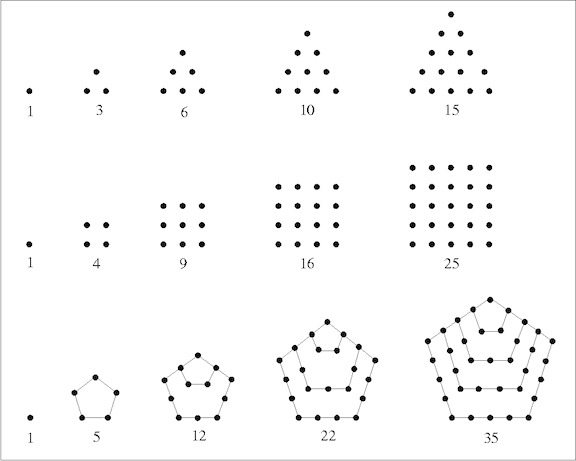# Perfect APThere is an arithmetic progression whose first term is 36, and the sum of the first $n$ terms of the AP is a perfect square for all positive integers $n$.

What is the 100th term of this arithmetic progression?

×